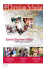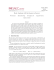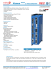# CAS CS 538. Solutions to Problem Set 6

## Transcription

CAS CS 538. Solutions to Problem Set 6
```BU CAS CS 538. Fall 2014.
1
CAS CS 538. Solutions to Problem Set 6
Problem 1. Suppose R has probability of success ε. We will use R to build a distinguisher D
between an encryption of m0 and an encryption of m1 (for any m0 6= m1 ) as follows. On input PK,
run R(PK) to get s; test if s = SK. If so, then upon receipt of a ciphertext c, decrypt it using s and
output 0 if the result is equal to m0 , and 1 otherwise. If not, output 0 or 1 randomly.
= Pr[D(Enc(m1 )) → 1] − Pr[D(Enc(m0 )) → 1]
= Pr[D(Enc(m1 )) → 1] − (1 − Pr[D(Enc(m0 )) → 0]) .
Let us consider just one term in this formula.
Pr[D(Enc(m1 )) → 1] = Pr[D(Enc(m1 )) → 1|s = SK] · Pr[s = SK]
+Pr[D(Enc(m1 )) → 1|s 6= SK] · Pr[s 6= SK]
1
= ε + (1 − ε)
2
Same for Pr[D(Enc(m0 )) → 0]. Plugging this into the above formula, we get that the advantage
of D is ε + 12 (1 − ε) + ε + 21 (1 − ε) − 1 = ε.
Problem 2. Just like in the Lamport scheme, we let SK = {x0 , x1 , x2 , x3 , x4 , x5 } and PK =
{y0 , y1 , y2 , y3 , y4 , y5 }, where yi = f (xi ) for a one-way function f . We will let each
of the 20 messages
correspond to one of the three-element subsets of {0, 1, 2, 3, 4, 5} (there are 63 = 20 such subsets).
It doesn’t matter how this correspondence is chosen: just build a table. A signature on a message
m consists of the three values xi for i ∈ S, where S is the subset corresponding to m. To verify
a signature consisting of three x values, check that f (xi ) = yi for i ∈ S, where, again, S is the
subset corresponding to m. Note that Lamport’s signature does the same thing, but uses only some
three-element subsets instead of all of them. The important thing for security is that no subset be
contained in another subset: that way, a potential forger is always required to invert the one-way
function for at least one element of the key.
Problem 3.
Lemma 1. For any x ∈ Z∗p , if x ∈ QR p , then −x 6∈ QR p . And if x 6∈ QR p , then −x ∈ QR p .
Proof. x ∈ QR p means that x(p−1)/2 = 1 (by problem set 2). So (−x)(p−1)/2 = (−1)(p−1)/2 x(p−1)/2 =
(−1)q · 1 = −1, because q is odd. So −x 6∈ QR p . The converse is proven the same way.
First, observe that the domain of β has size (p − 1)/2 = q, because exactly half of Z∗p are squares.
The range of β also has size q. Thus, if we show that β is one-to-one, we will have shown that it is
a bijection. So we need to show that for any pair a 6= b, β(a) 6= β(b). Indeed, if a ≤ q and b ≤ q, or
a > q and b > q, this is trivial. If a ≤ q and b > q, then we need to show that p − b 6= a. We know
that b ∈ QR p , so p − b = −b 6∈ QR p by the above Lemma; but a ∈ QR p , so p − b cannot be equal to
a. Same if a > q and b ≤ q.
BU CAS CS 538. Fall 2014.
2
The forward direction is trivially efficiently computable. The reverse direction is efficiently computable as follows. Let z ∈ 1, . . . , q by some value. We want to compute β −1 (z). First, check if
z ∈ QR p (recall from problem set 2 problems 1(e)(f) that testing whether z ∈ QR p can be done
efficiently by raising z q mod p and seeing if it is equal to 1 or −1). If so, output z (this is the correct
inverse, because it is in QR p and because z ≤ q, so β(z) = z). Else, output p − z (this is the correct
inverse, because p − z ∈ QR p by the above lemma and p − z > q, so β(p − z) = z).
Now, take the hash function from class (the that computes H(a, b) = g a hb mod p and modify it
by computing H 0 (a, b) = β(g a hb mod p). I claim that if H is collision resistant, then H 0 is collision
resistant. Indeed, the reduction is trivial: if an adversary, given (p, g, h), can output a, b, c, d such that
H 0 (a, b) = H 0 (c, d), then H(a, b) = H(c, d) (because β is a bijection), so the same exact adversary
can be used to break the collision resistance of H.
Problem 4. Fix p and a generator g of QR p . On input x, y ∈ {1, 2, . . . q}, the PRG outputs
a = β(g x ), b = β(g y ), c = β(g xy ). Suppose our PRG is insecure: that is, there is a distinguisher D
that can tell a, b, c from three random elements of {1, 2, . . . , q} with some non negligible advantage
. We will build a distinguisher D0 to violate the DDH assumption. Recall that he DDH assumption
says that the three values g x , g z , g xy are indistinguishable from three uniformly random elements of
QR p . We will build D0 as follows: apply β to each of the three inputs and give the result to D0 .
If the three inputs are a Diffie-Hellman triple, then the result is distributed the exact same way as
(a, b, c). If they are random, the the result is three random elements of {1, 2, . . . q}. Therefore, the
distinguishing advantage of D0 is also , which is a contradiction to the DDH assumption.
```

### Library Resources (PDF, > 1MB)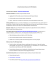### COURSE OUTLINE Fall 2014 1. Calendar Information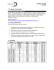### CITY OF LOS ANGELES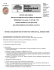### Wednesday, October 22, 2014 - ENC General Meeting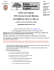### 202A: Solutions to Homework V Piotr Achinger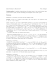### Universally Composable Security with Specialized Environments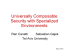### Telchart Electronic Chart Display and Information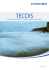### Homework 2 Out: 09/26/14 Due: 10/10/14 11:59pm U M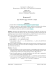### Document 6498654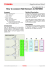### PDF report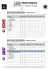### PDF Match Report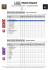### Sample file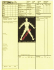### 1 Window Jumbo Strip Game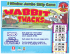### Christian Scholar Fall 2012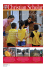### Christian Scholar Fall 2009